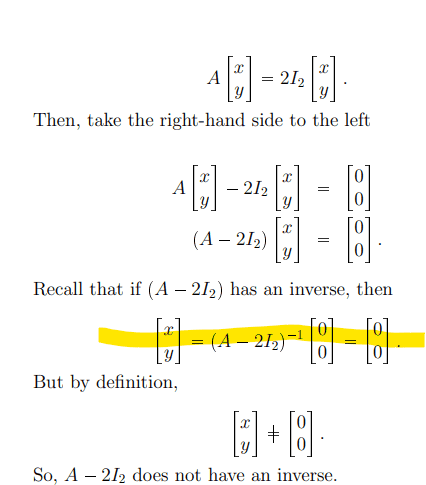# Proving eigenvalues of a 2 x 2 square matrix

• ChiralSuperfields

#### ChiralSuperfields

Homework Statement
Relevant Equations
For this,Does someone please know why the equation highlighted not be true if ##(A - 2I_2)## dose not have an inverse?

Many thanks!

For this,
View attachment 327021
Does someone please know why the equation highlighted not be true if ##(A - 2I_2)## dose not have an inverse?

Many thanks!
You “take” the matrix to the other side of the equation by multiplying from the left each side of the equation by the inverse. If the inverse does not exist, one cannot multiply by it.

•FactChecker and ChiralSuperfields
Eigenvalues ##\lambda## for a matrix ##A##are defined to satisfy ##Det(A-\lambda I)=0##. This comes from ##Ax=\lambda x ##, so that ##(A-\lambda I )x=0 ##.

•ChiralSuperfields
@ChiralSuperfields, what textbook are you getting this stuff from? Your thread here seems related to two of you recent threads. As I mentioned before, finding eigenvalues of a matrix has nothing to do with finding the inverse of a matrix.

The definition of an eigenvalue (usually represented by ##\lambda##) is that for some specific vector ##\vec x##, ##A\vec x = \lambda \vec x##, or equivalently, ##(A - \lambda I)\vec x = \vec 0##. If we restrict ##\vec x## to nonzero vectors, it must be true that ##|A - \lambda I| = 0##. That means that ##A - \lambda I## does not have an inverse.

One other thing. Near the bottom of the attachment you posted it says
But by definition,
##\begin{bmatrix}x \\ y \end{bmatrix} \ne \begin{bmatrix}0 \\ 0 \end{bmatrix}##

Unless it was specifically stated that this vector was nonzero somewhere above what you posted in the attachment, the line I quoted makes no sense.

•ChiralSuperfields and Steve4Physics
@ChiralSuperfields, what textbook are you getting this stuff from? Your thread here seems related to two of you recent threads. As I mentioned before, finding eigenvalues of a matrix has nothing to do with finding the inverse of a matrix.

The definition of an eigenvalue (usually represented by ##\lambda##) is that for some specific vector ##\vec x##, ##A\vec x = \lambda \vec x##, or equivalently, ##(A - \lambda I)\vec x = \vec 0##. If we restrict ##\vec x## to nonzero vectors, it must be true that ##|A - \lambda I| = 0##. That means that ##A - \lambda I## does not have an inverse.

One other thing. Near the bottom of the attachment you posted it says

Unless it was specifically stated that this vector was nonzero somewhere above what you posted in the attachment, the line I quoted makes no sense.
Thank you for your replies @Frabjous , @WWGD , and @Mark44!

I understand now :) @Mark44, this is not from a textbook but course notes.

Many thanks!

this is not from a textbook but course notes.
It's hard to tell where they're going with these notes.

•ChiralSuperfields